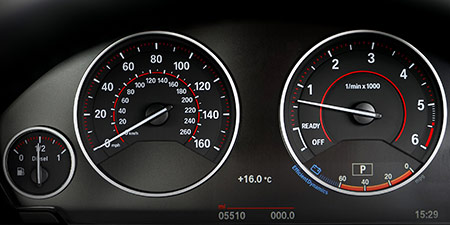# Speed of light to Miles per second (mi/s)

Units of speed ﹣ Converter Speed of light to Miles per second

Here you can convert the Speed unit Speed of light into the unit Miles per second and vice versa you can convert Miles per second into Speed of light. By clicking the "Swap units" icon, you will always obtain the desired conversion in the calculation result. With the following calculator you can also calculate any other Speed unit.

## Info about "Speed of light"

Definition: If an object moves at the light speed for one second, it travels a distance of 299,792.458 km or about 300,000 km. This is the light speed that light travels in a vacuum.

This speed is also used to propagate radio signals, which is very important in space travel. For example, a signal from Mars takes about 3 to 20 minutes to reach Earth depending on its actual position. We notice a sunrise on earth about 8 minutes later because the light of the sun arrives to our eyes at that time.

## Info about "Miles per second"

The length unit "mile" is commonly used in the Anglo-American countries, where speeds are represented in miles (instead of kilometers) per time unit and it should not be confused sea mile.

The units are defined as follows: An object moving at 1 "mph" per hour will cover a distance of one mile. Therefore, 1 mile is equivalent to 1.609344 kilometers.

## Formula for the conversion of Speed of light to Miles per second (mi/s) and vice versa

The calculation from Speed of light to Miles per second shall be made using the following conversion formula:

Conversion formula Speed of light to Miles per second

Determine the number of Miles per second from Speed of light

Speed of light × 186282.39705122

## Formula for the conversion of Miles per second (mi/s) to Speed of light

The calculation from Miles per second to Speed of light shall be made using the following conversion formula:

Conversion formula Miles per second to Speed of light

Determine the number of Speed of light from Miles per second

Miles per second × 5.3681937522257E-6

## Overview table : How many Speed of light are in a Miles per second ?

Speed of light ⇒ Miles per second mi/s
0.01   are  1 862.82397 mi/s
0.02   are  3 725.64794 mi/s
0.03   are  5 588.47191 mi/s
0.04   are  7 451.29588 mi/s
0.05   are  9 314.11985 mi/s
0.06   are  11 176.94382 mi/s
0.07   are  13 039.76779 mi/s
0.08   are  14 902.59176 mi/s
0.09   are  16 765.41573 mi/s
0.10   are  18 628.23970 mi/s
0.20   are  37 256.47941 mi/s
0.30   are  55 884.71911 mi/s
0.40   are  74 512.95882 mi/s
0.50   are  93 141.19852 mi/s
0.60   are  111 769.43823 mi/s
0.70   are  130 397.67793 mi/s
0.80   are  149 025.91764 mi/s
0.90   are  167 654.15734 mi/s
1   corresponds to  186 282.39705 mi/s
2   are  372 564.79410 mi/s
3   are  558 847.19115 mi/s
4   are  745 129.58820 mi/s
5   are  931 411.98525 mi/s
6   are  1 117 694.38230 mi/s
7   are  1 303 976.77935 mi/s
8   are  1 490 259.17641 mi/s
9   are  1 676 541.57346 mi/s
10   are  1 862 823.97051 mi/s
20   are  3 725 647.94102 mi/s
30   are  5 588 471.91153 mi/s
40   are  7 451 295.88204 mi/s
50   are  9 314 119.85256 mi/s
60   are  11 176 943.82307 mi/s
70   are  13 039 767.79358 mi/s
80   are  14 902 591.76409 mi/s
90   are  16 765 415.73461 mi/s
100   are  18 628 239.70512 mi/s
200   are  37 256 479.41024 mi/s
300   are  55 884 719.11536 mi/s
400   are  74 512 958.82048 mi/s
500   are  93 141 198.52561 mi/s
600   are  111 769 438.23073 mi/s
700   are  130 397 677.93585 mi/s
800   are  149 025 917.64097 mi/s
900   are  167 654 157.34609 mi/s
1 000   are  186 282 397.05122 mi/s

## Overview table : How many Miles per second are in a Speed of light ?

Miles per second mi/s ⇒ Speed of light
0.01 mi/s  are  0.0000000536
0.02 mi/s  are  0.0000001073
0.03 mi/s  are  0.0000001610
0.04 mi/s  are  0.0000002147
0.05 mi/s  are  0.0000002684
0.06 mi/s  are  0.0000003220
0.07 mi/s  are  0.0000003757
0.08 mi/s  are  0.0000004294
0.09 mi/s  are  0.0000004831
0.10 mi/s  are  0.0000005368
0.20 mi/s  are  0.0000010736
0.30 mi/s  are  0.0000016104
0.40 mi/s  are  0.0000021472
0.50 mi/s  are  0.0000026841
0.60 mi/s  are  0.0000032209
0.70 mi/s  are  0.0000037577
0.80 mi/s  are  0.0000042945
0.90 mi/s  are  0.0000048313
1 mi/s  corresponds to  0.0000053681
2 mi/s  are  0.0000107363
3 mi/s  are  0.0000161045
4 mi/s  are  0.0000214727
5 mi/s  are  0.0000268409
6 mi/s  are  0.0000322091
7 mi/s  are  0.0000375773
8 mi/s  are  0.0000429455
9 mi/s  are  0.0000483137
10 mi/s  are  0.0000536819
20 mi/s  are  0.0001073638
30 mi/s  are  0.0001610458
40 mi/s  are  0.0002147277
50 mi/s  are  0.0002684096
60 mi/s  are  0.0003220916
70 mi/s  are  0.0003757735
80 mi/s  are  0.0004294555
90 mi/s  are  0.0004831374
100 mi/s  are  0.0005368193
200 mi/s  are  0.0010736387
300 mi/s  are  0.0016104581
400 mi/s  are  0.0021472775
500 mi/s  are  0.0026840968
600 mi/s  are  0.0032209162
700 mi/s  are  0.0037577356
800 mi/s  are  0.0042945550
900 mi/s  are  0.0048313743
1 000 mi/s  are  0.0053681937